# Texas Go Math Grade 7 Lesson 4.4 Answer Key Ratios and Pi

Refer to our Texas Go Math Grade 7 Answer Key Pdf to score good marks in the exams. Test yourself by practicing the problems from Texas Go Math Grade 7 Lesson 4.4 Answer Key Ratios and Pi.

## Texas Go Math Grade 7 Lesson 4.4 Answer Key Ratios and Pi

Exploring Circumference
A circle is a set of points in a plane that are a fixed distance from the center.

A radius is a line segment with one endpoint at the center of the circle and the other endpoint on the circle. The length of a radius is called the radius of the circle.

A diameter of a circle is a line segment that passes through the center of the circle and whose endpoints lie on the circle. The length of the diameter is twice the length of the radius. The length of a diameter is called the diameter of the circle.

The circumference of a circle is the distance around the circle.A. Use a measuring tape to find the circumference of five circular objects. Then measure the distance across each item to find its diameter. Record the measurements of each object in the table below.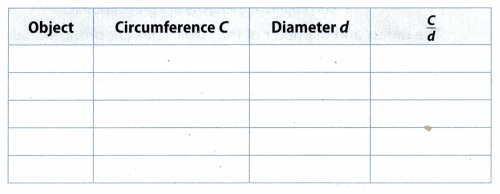B. Divide the circumference of each object by its diameter. Round your answer to the nearest hundredth.

Reflect

Question 1.
Make a Conjecture Describe what you notice about the ratio $$\frac{C}{d}$$ in your table.
All, ratios when calculated are approximately 3.14.

Question 2.
In every circle, what is the ratio of the radius to the diameter? What is the ratio of the circumference to the diameter?
In every circle, the ratio of the radius to the diameter is 1 : 2
The ratio of the circumference to the diameter is π

Question 3.
Draw Conclusions Based on the ratios of the radius to diameter and circumference to diameter, are all circles proportional? Is the ratio of circumference to the diameter of any circle the same for all circles?
Yes, all circles are proportional based on the ratios of the radius to diameter and circumference to diameter.
The ratio of circumference to the diameter of any circle will be equal to π and same for all circles.

Question 4.
Determine if the radius and diameter of the two circles are proportional.Find the radius and diameter of each circle
First circle:
r1 = 8 ft
d1 = 2 × r= 2 × 8= 16 ft
Second circLe:
d2 = 9 ft
r2 = d ÷ 2 = 9 ÷ 2 = 4.5 ft
Set up a proportion using the corresponding lengths of the radius and diameterThe radius and diameter of the two circles are proportional.

Question 5.
The circumference of the larger circle is approximately 44 meters and the circumference of the smaller circle is approximately 11 meters. Use a proportion to find the approximate
diameter of the smaller circle.__________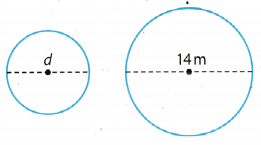C2 = 44 m
C1 = 11 m
d2 = 14 m
Set up a proportion using the given information for the larger and smaller circle.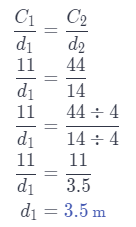Fill in the blanks. (Explore Activity)

Question 1.
Vocabulary In any circle, the ratio of the ___________ to the diameter is pi.
In any circle, the ratio of the circumference to the diameter is pi.

Question 2.
Vocabulary In any circle, the ratio of the ____________ radius is 2.
In any circle, the ratio of the diameter to the radius is 2.

Question 3.
You can use the decimal number ________ or the fraction ______ as an approximation for pi.
You can use the decimal number 3.14 or the fraction $$\frac{C}{d}$$ as an approximation for pi.

Question 4.
Determine if the radius and diameter of a circle with a diameter of 100 mm and a circle with a diameter of 10 mm are proportional. (Example 1)
Find the radius and diameter of each circle.
First circle:
d1 = 100 mm
r1 = d ÷ 2 = 100 ÷ 2 = 50 mm
Second circle:
d2 = 10 mm
r2 = d ÷ 2 = 10 ÷ 2 = 5 mm
Set up a proportion using the corresponding Lengths of the radius and diameter.
$$\frac{50}{5} \stackrel{?}{=} \frac{100}{10}$$
10 = 10
The radius and diameter of the two circles are proportional.

Question 5.
Is the circle represented by a penny similar to the one of a quarter? Explain. (Example 1)
Every circle is proportional to each other. Thus, the circle represented by a penny is similar to the one of a quarter.

Question 6.
The circumference of the larger circle is about 18.8 centimeters and the circumference of the smaller circle is about 6.3 centimeters Find the approximate diameter of the smaller circle. (Example 2)C2 = 18.8 cm
C1 = 6.3 cm
d2 = 6 m
Set up a proportion using the given information for the larger and smaller circle.
$$\frac{C_{1}}{d_{1}}=\frac{C_{2}}{d_{2}}$$
$$\frac{6.3}{d_{1}}=\frac{18.8}{6}$$
18.8d1 = 6.3 × 6
18.8d1 = 37.8
d1 = 37.8 ÷ 18.8
d1 ≈ 2 cm

Essential Question Check-In

Question 7.
What is the result of dividing the distance around a circle by the distance across the same circle? What number can you use as an approximate value for this ratio?
The result of dividing the distance around a circle by the distance across the same circle is π(pi).
We can use the decimal number 3.14 to approximate value for π.

Question 8.
Measurement Jillian measured the distance around a small fishpond as 27 yards. Which would be a good estimate for the distance across the pond, 14 yards, 9 yards, or 7 yards? Explain how you decided.
The distance around a small fishpond is the circumference(C).
The distance around the pond is the diameter(d).
C = 27 yd
We know that $$\frac{C}{d}$$ ≈ 3.14
$$\frac{C}{d}$$ ≈ 3.14
$$\frac{27}{d}$$ ≈ 3.14
3.14d ≈ 27
d ≈ 27 ÷ 3.14
d ≈ 8.6 yd
A good estimate for the distance across the pond would be 9 yards.

Question 9.
A rotating wind turbine has a diameter of about 185 feet and its circumference is about 580 feet. A smaller model of the turbine has a circumference of about 10 feet. What will the diameter of the model be?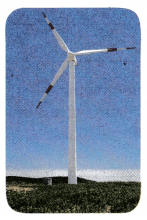d1 = 185 ft
C1 = 580 ft
C2 = 10 ft
Set up a proportion using the given information for the larger and smaller wind turbineThe diameter of the model will be 319 ft

Question 10.
Multistep Andrew has a flying disc with a radius of 10 centimeters. What is the circumference of the disc? (Remember $$\frac{C}{d}$$ = 3.14.)
r = 10 cm ⇒ d = 20 cm
Now, calcuLate the circumference of the disc using that $$\frac{C}{d}$$ = 3.14
$$\frac{C}{d}$$ = 3.14
$$\frac{C}{20}$$ = 3.14
C = 20 × 3.14
C = 62.8 cm
The circumference of the disk is 62.8 cm.

Question 11.
Mandie wants to put some lace trim around the outside of a round tablecloth she expanded. The original tablecloth had a radius of 2 feet and a circumference of 12.56 feet. If the tablecloth now has a radius of 3 feet, is 15 feet of lace enough? Explain.
Calculate the circumference of the new tablecloth to find out if 15 feet of lace is enough.
r1 = 2 ft ⇒ d1 = 4 ft
C1 = 12.56 ft
r2 = 3 ft ⇒ d2 = 6 ft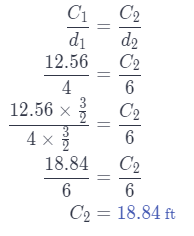Since the circumference of the new tablecloth is 18.86 ft which is greater than 15 ft, 15 feet of lace is not enough to put around the outside of the new tablecloth.

Question 12.
Marta is making two different charms for a necklace. One charm has a 1 centimeter diameter and a 3.14 centimeter circumference. A similar charm has a diameter of 4 centimeters. What is the circumference?
C = 3.14 cm The circumference of charm
d = 1 cm Diameter of charm
C1 = ? The circumference of simiLar charm
d1 = 4 cm Diameter of similar charm
Use a proportion to find the value of the circumference of the similar charm.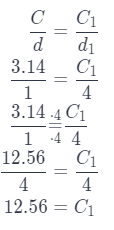The circumference of the similar charm is 12.56 cm.

Question 13.
Randy is putting bricks around the outside of his round flower bed to protect the plants.
a. If the diameter of his flower bed is 100 inches, what is the distance around the garden? (Remember $$\frac{C}{d}$$ = 3.14.)
To find the distance around the garden, we have to find the circumference of the round flower bed
Use the formula for circumference of the circle
C = π(d) Substitute 100 for d and 3.14 for π.
C ≈ 3.14 ∙ 100
C ≈ 314
The distance around the garden is about 314 in.

b. If the curved bricks he wants to buy are each half a foot long, how many will he need to put around the outside of the garden? Explain.
First, convert inches to feet
1 in. = 0.083 ft
C ≈ 314 ∙ 0.83 = 26.26ft
The circumference of the flower bed is 26.26 ft
One brick is half a feet Long, se we have to divide C by $$\frac{1}{2}$$ to find how many bricks will he need to buy.
26.06 ÷ $$\frac{1}{2}$$ = 26.06 ∙ 2 = 52.12
Randy needs 52.12 bricks to put around the outside of the garden.

c. If each brick costs $0.68, and he can only buy whole bricks, how much will it cost him to get the material to put around the outside of his garden? Answer: He needs 52.12 bricks, so he must buy 53 whole bricks. One brick costs$0.68. therefore
The cost of whole material 0.68 ∙ 53 = 36.04
The material will cost him $36.04. d. If his mother decides he can only have half of that diameter for his flower bed, how will the cost of the bricks be affected? Explain? Answer: The circumference of the flower bed with half of the diameter is half of the circumference with the whole diameter. Hence, he will need half of the number of the bricks, so the cost of the bricks will be halfed. $$\frac{36.04}{2}$$ =$18.02

Question 14.
Your grandmother is teaching you how to make a homemade pie. The pie pan has a diameter of 9 inches.
a. If she asks you to cut a strip of pie crust long enough to go around the outside of the pan, how long does it need to be? (Remember $$\frac{C}{d}$$ = 3.14.)
C = ? The circumference of pan
d = 9 in Diameter of pan
Use a equation $$\frac{C}{d}$$ = 3.14 to find a value of circumference. Substitute value for diameter.
$$\frac{C}{9}$$ = 3.14
Multiply both sides by 9.
9 ∙ $$\frac{C}{9}$$ = 9 ∙ 3.14
C = 28.26
It need to be 28.26 inches long.

b. If another pie pan is 8 inches across the diameter, how long does that piece of crust need to be?
C = ? The circumference of pan
d = 8 + 9 = 17 in Diameter of pan
Use a equation $$\frac{C}{d}$$ = 3.14 to find a value of circumference. Substitute value for diameter.
$$\frac{C}{17}$$ = 3.14
Multiply both sides by 17.
17 ∙ $$\frac{C}{17}$$ = 17 ∙ 3.14
C = 53.38
It need to be 53.38 inches Long.

H.O.T. Focus on Higher Order Thinking

Question 15.
Make a Conjecture You know that all squares are similar and all circles are similar. An equilateral triangle has 3 equal sides and 3 angles of 60 degrees each. Are all equilateral triangles similar?
Yes they are, because the corresponding angles of all equilateral triangles are equal, and corresponding sides are proportional.

Question 16.
Multiple Representations You know three different number representations for pi that you can use to approximate the answer to a problem. Describe a situation when you might choose to use $$\frac{22}{7}$$.
You might choose to use $$\frac{22}{7}$$ when you have diameter which value is 7.
For example:
Find the circumference of circle if diameter is 7 centimeters.
C = ? The circumference of circle
d = 7 cm Diameter of circle
Use a equation $$\frac{C}{d}$$ = $$\frac{22}{7}$$ to find a value of circumference Substitute value for diameter.
$$\frac{C}{7}$$ = $$\frac{22}{7}$$
Therefore C = 22 centimeters.

Question 17.
Represent Real-World Problems Describe an example in your daily life where you might be able to measure around something but could not measure across it.
One example is that when you want to measure the circumference of a post. We could use a measuring tool to determine the circumference of the post by simply going around the post. However, if we want to determine the area of the post, it is somewhat impossible. First, the post was already attached to the ground and depending on the height of the post, we could not check the diameter or radius of the post.

Another one is measuring the circumference and area of a tree. We could find the circumference of a tree by simply going around the tree. But if you want to measure the area of a tree, it is impossible unless you cut the tree to determine the diameter or radius of the tree.

You can measure the circumference of a post or tree but you can’t measure their area.

Question 18.
Critical Thinking Every morning Jesse runs 3 laps on a circular track. One morning the track is closed, but the straight path from one side of the track to the other is open. How many times should Jesse run across the path if he wants to run his usual distance? Explain your answer.
Therefore, if you want to calculate how much times he must run across the path to run his usual distance, use formula $$\frac{C}{d}$$ = 3.14 to find C.